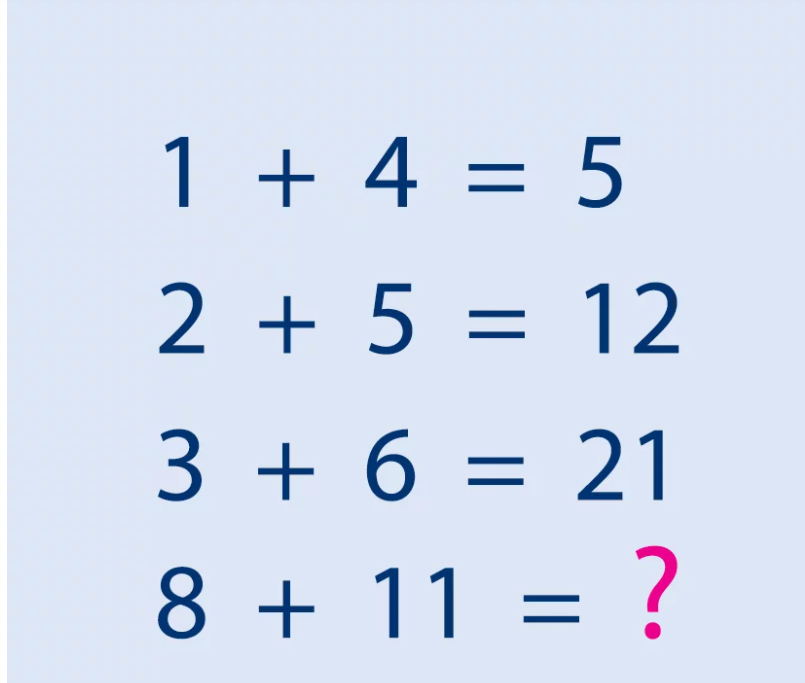# Can You Guess the Two Answers to This Viral IQ Test?

#### ByLilit

Jul 10, 2021Test your knowledge with this tricky math equation. Can you give your brain a little exercise today? Keep that mid-week slump at bay by trying to solve a math problem that’s been showing up everywhere. Typically math equations have one correct solution, but this particular problem is an exception.

There are two different ways to solve the puzzle, and each method will give you a different answer. Its interesting that both are correct, but both are pretty tricky to figure out.The First Solution: For the first line, add 1 to 4 to get 5. Moving onto the next line, add 2 and 5 to the previous solution (5) for a total of 12. The third line follows that pattern, meaning that you add 3 + 6 + 12 to get 21. Which means that, for the final line, you would add 8 + 11 + 21 to get a grand total of 40. Didn’t get 40?Lest find the second way to solve the problem.

The Second Solution: The second method, involves adding together the first number, and the first number times the second number. To get 5 for the first line, you add 1 to 1 times 4. Similarly, the second line would be 2 + 2(5) = 12. For the third line, add 3 + 3(6) to get 21. Using this method for the final line, add 8 + 8(11) to get a final answer of 96.